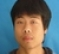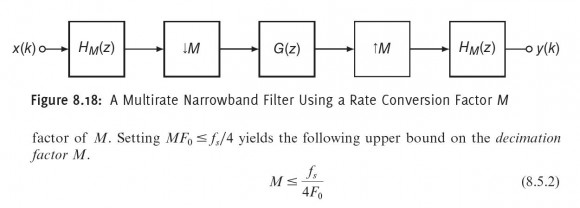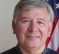## Upper bound on the decimation factor MStarted by 6 years ago8 replieslatest reply 6 years ago154 views

Dear experts,

Hi, I am a Chinese student at BeiHang Uni. I am now self-studying DSP. I had a difficulty understanding a restraint on the #Decimation factor M in #Multirate Narrowband Filter.I don't understand why decimation factor must satisfy (8.5.2). I read an article which writes it's to achieve gain in computational efficiency(http://www.mds.com/wp-content/uploads/2014/05/multirate_article.pdfpage 9)  and I still had no clue. Could you give me a hint ?

[ - ]Computational efficiency comes from two places - when you down sample you can skip over blocks of data so you only apply your filter every M samples and second from the number of taps you need to create the filter.  The bigger M is, the fewer computations per input samples you need to do.  But if $F_0$ is really small, the number of taps is large.  So I think there has to be a balance in there.

If M is big, you only have to do a filter step once every M input samples, so it saves you computation effort.  The best thing to do is to create an example - build a simple filter and change a few parameters so you can see what the math really tells you. Send in a simple square wave and see what comes out of the filter.  Change the parameters, and compare what comes out.  It should not take long to understand the formulas after that!

Dr. mike

[ - ]Thank you, Dr. mike!!!

[ - ]In the article at the link you provided, there is an expression:

"To achieve gain in computational efficiency, the following must hold:B<Fs/4"

I'm sure you know that B<Fs/2 is just the Nyquist constraint.  And, here, you want to reduce the bandwidth to allow a lower sample rate.  Factors of 2 are the most common in doing this.  So, the first decimation factor of 2 yields B<Fs/4.

[ - ]Dr. mike, Do you agree with me on this? I think the upper bond is fs/2F0 for M. Here are my reasons.

Here fs stands for sampling rate, F0 is the cutoff frequency of the narrowband filter which is fixed and needs to meet.

After down sampling, the sampling is fs/M, so if the bandwidth of the signal is lower than the fs/2M, it won't cause aliasing. Therefore, a lowpass filter with a cutoff frequency, F0, less than fs/2M is possible. From F0<fs/2M, I get M<fs/2F0, twice of the upper bound in the book.

Do I make sense?

[ - ]Making sense can also be wrong :-)  It is possible you are right, and the book has a typo - that happens to every author.  But there are 2 steps in the filter process, one is down sample and one is up sample.  I suspect the other factor of 2 comes from the desire to prevent artefacts from appearing in the up sample spectrum.  If all you were doing is changing rate down, then you would be correct.

Try it.  Build a filter and feed in a square wave.  If you get a sine wave out, the filter works.  If you get noise - you violated a condition and that extra factor of 2 is there for a reason.

Dr. mike

[ - ]The document(page 9) says B to be Fs/4 for design to be more efficient than using a single rate filter.

imagine B = 0.5 Fs (M = 1, i.e. no decimation)

B = 0.4Fs(M = 2, not efficient relative to single rate filter)

B = 0.125Fs (M = 4, efficiency starts here)

B = 0.0625 (M = 8, better)

an so on.

Kaz

[ - ]Thank you for your time. But how can the second not efficient relative to single rate filter? Could you shed some lights on this?

Chuan

[ - ]Correction:

B = 0.5(M = 1, no decimation possible)

B = 0.25Fs(M = 2, not efficient relative to single rate filter)

B = 0.125Fs (M = 4, efficiency starts here)

B = 0.0625 (M = 8, better)

The author has compared the efficiency and came out with some figures. There is really nothing rock solid here.

I assume M to be integer 4 or more to be useful. Remember we are comparing one filter (single rate case) with two filters (multirate case).

So try your own study with various B and various filter spec to see the difference.

Kaz